# Lec 1 introduction surveying by et bilal

16. Oct 2016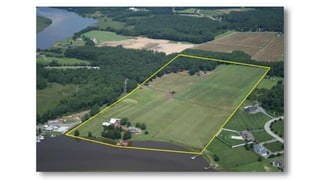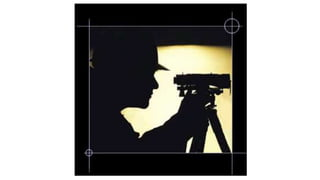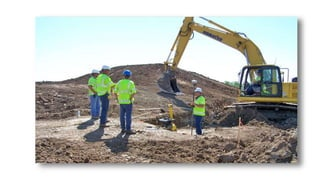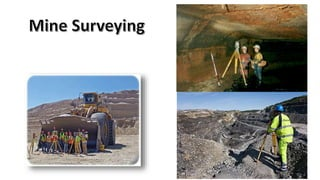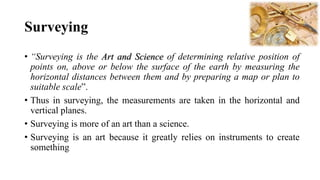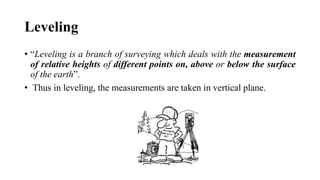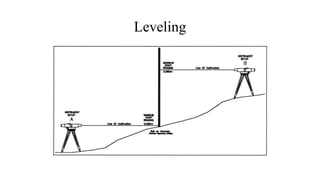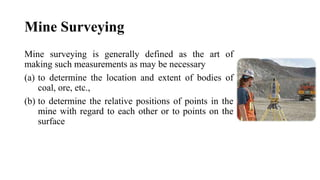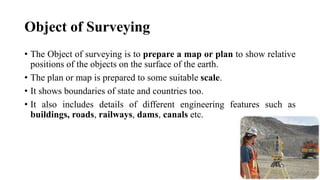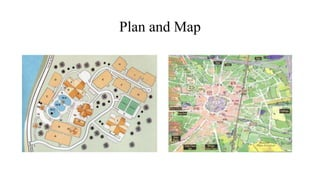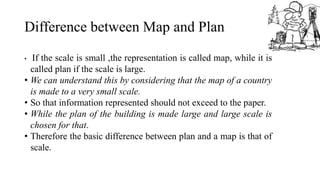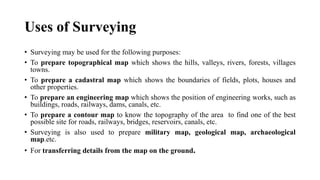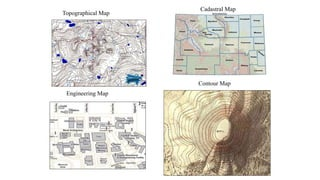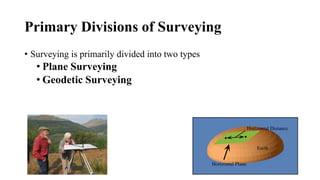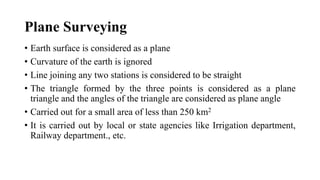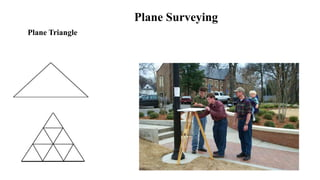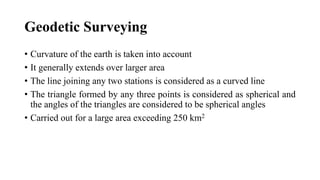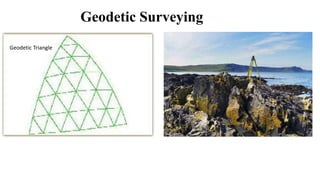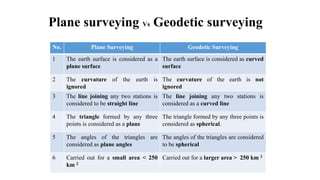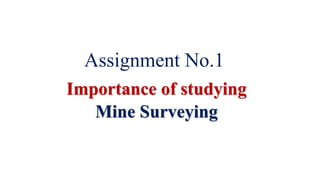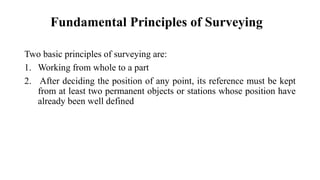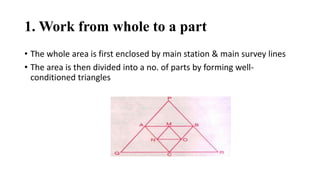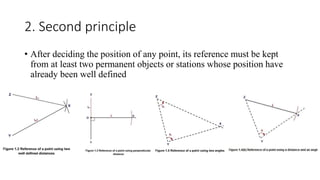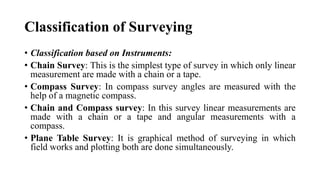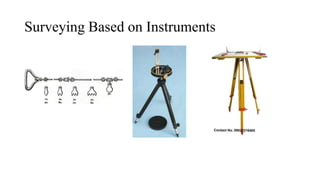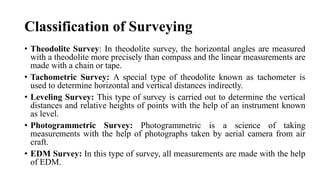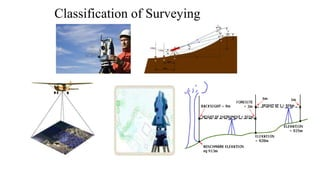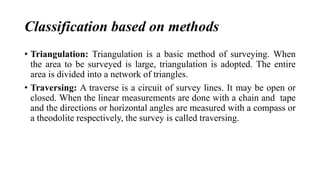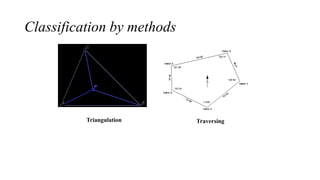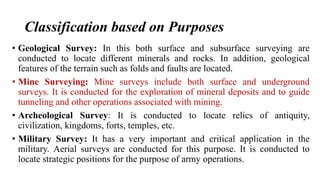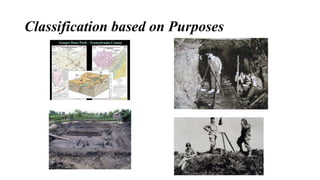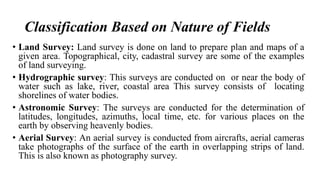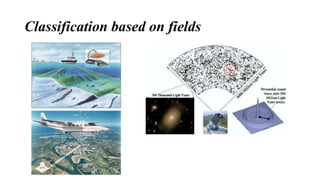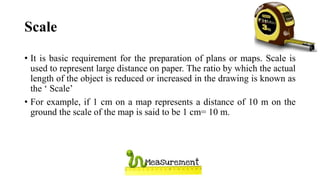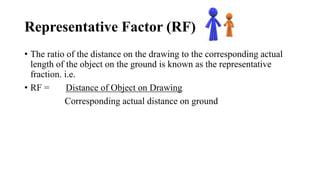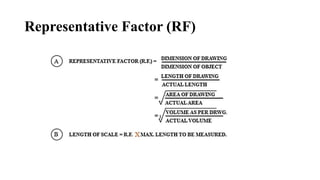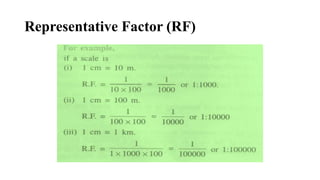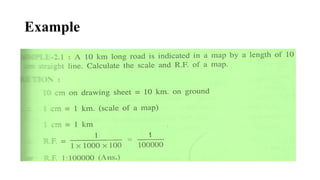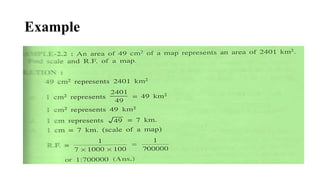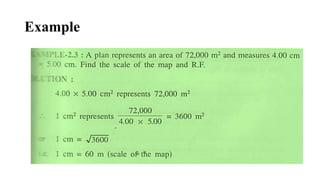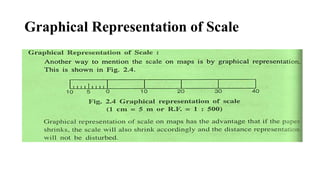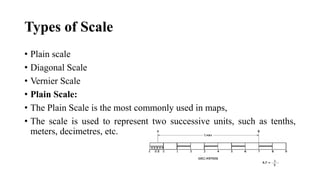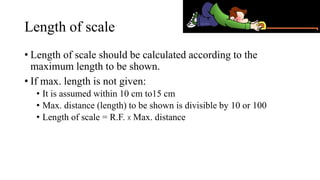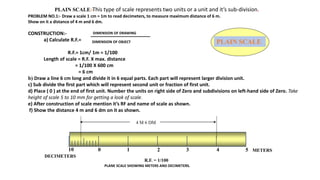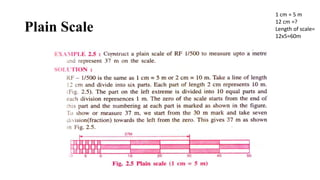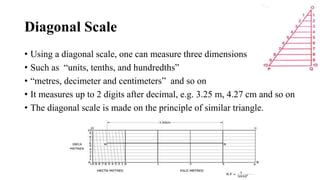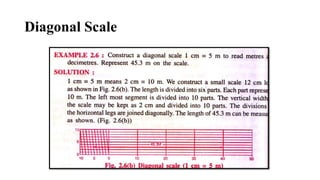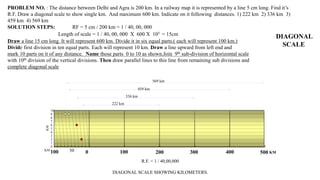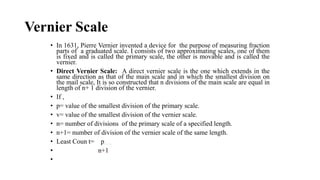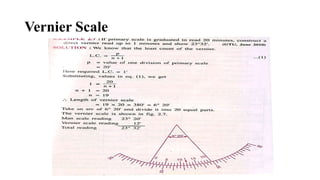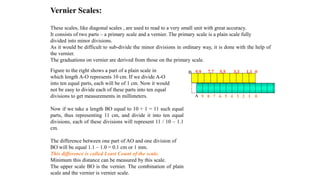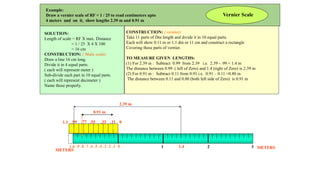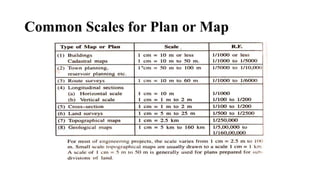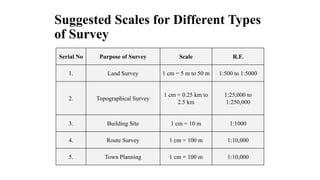1 von 56

### Lec 1 introduction surveying by et bilal

• 5. Surveying • “Surveying is the Art and Science of determining relative position of points on, above or below the surface of the earth by measuring the horizontal distances between them and by preparing a map or plan to suitable scale”. • Thus in surveying, the measurements are taken in the horizontal and vertical planes. • Surveying is more of an art than a science. • Surveying is an art because it greatly relies on instruments to create something
• 6. Leveling • “Leveling is a branch of surveying which deals with the measurement of relative heights of different points on, above or below the surface of the earth”. • Thus in leveling, the measurements are taken in vertical plane.
• 8. Mine Surveying Mine surveying is generally defined as the art of making such measurements as may be necessary (a) to determine the location and extent of bodies of coal, ore, etc., (b) to determine the relative positions of points in the mine with regard to each other or to points on the surface
• 9. Object of Surveying • The Object of surveying is to prepare a map or plan to show relative positions of the objects on the surface of the earth. • The plan or map is prepared to some suitable scale. • It shows boundaries of state and countries too. • It also includes details of different engineering features such as buildings, roads, railways, dams, canals etc.
• 11. Difference between Map and Plan • If the scale is small ,the representation is called map, while it is called plan if the scale is large. • We can understand this by considering that the map of a country is made to a very small scale. • So that information represented should not exceed to the paper. • While the plan of the building is made large and large scale is chosen for that. • Therefore the basic difference between plan and a map is that of scale.
• 12. Uses of Surveying • Surveying may be used for the following purposes: • To prepare topographical map which shows the hills, valleys, rivers, forests, villages towns. • To prepare a cadastral map which shows the boundaries of fields, plots, houses and other properties. • To prepare an engineering map which shows the position of engineering works, such as buildings, roads, railways, dams, canals, etc. • To prepare a contour map to know the topography of the area to find one of the best possible site for roads, railways, bridges, reservoirs, canals, etc. • Surveying is also used to prepare military map, geological map, archaeological map.etc. • For transferring details from the map on the ground.
• 14. Primary Divisions of Surveying • Surveying is primarily divided into two types • Plane Surveying • Geodetic Surveying
• 15. Plane Surveying • Earth surface is considered as a plane • Curvature of the earth is ignored • Line joining any two stations is considered to be straight • The triangle formed by the three points is considered as a plane triangle and the angles of the triangle are considered as plane angle • Carried out for a small area of less than 250 km2 • It is carried out by local or state agencies like Irrigation department, Railway department., etc.
• 17. Geodetic Surveying • Curvature of the earth is taken into account • It generally extends over larger area • The line joining any two stations is considered as a curved line • The triangle formed by any three points is considered as spherical and the angles of the triangles are considered to be spherical angles • Carried out for a large area exceeding 250 km2
• 19. Plane surveying Vs Geodetic surveying No. Plane Surveying Geodetic Surveying 1 The earth surface is considered as a plane surface The earth surface is considered as curved surface 2 The curvature of the earth is ignored The curvature of the earth is not ignored 3 The line joining any two stations is considered to be straight line The line joining any two stations is considered as a curved line 4 The triangle formed by any three points is considered as a plane The triangle formed by any three points is considered as spherical. 5 The angles of the triangles are considered as plane angles The angles of the triangles are considered to be spherical 6 Carried out for a small area < 250 km 2 Carried out for a larger area > 250 km 2
• 20. Assignment No.1 Importance of studying Mine Surveying
• 21. Fundamental Principles of Surveying Two basic principles of surveying are: 1. Working from whole to a part 2. After deciding the position of any point, its reference must be kept from at least two permanent objects or stations whose position have already been well defined
• 22. 1. Work from whole to a part • The whole area is first enclosed by main station & main survey lines • The area is then divided into a no. of parts by forming well- conditioned triangles
• 23. 2. Second principle • After deciding the position of any point, its reference must be kept from at least two permanent objects or stations whose position have already been well defined
• 24. Classification of Surveying • Classification based on Instruments: • Chain Survey: This is the simplest type of survey in which only linear measurement are made with a chain or a tape. • Compass Survey: In compass survey angles are measured with the help of a magnetic compass. • Chain and Compass survey: In this survey linear measurements are made with a chain or a tape and angular measurements with a compass. • Plane Table Survey: It is graphical method of surveying in which field works and plotting both are done simultaneously.
• 25. Surveying Based on Instruments
• 26. Classification of Surveying • Theodolite Survey: In theodolite survey, the horizontal angles are measured with a theodolite more precisely than compass and the linear measurements are made with a chain or tape. • Tachometric Survey: A special type of theodolite known as tachometer is used to determine horizontal and vertical distances indirectly. • Leveling Survey: This type of survey is carried out to determine the vertical distances and relative heights of points with the help of an instrument known as level. • Photogrammetric Survey: Photogrammetric is a science of taking measurements with the help of photographs taken by aerial camera from air craft. • EDM Survey: In this type of survey, all measurements are made with the help of EDM.
• 28. Classification based on methods • Triangulation: Triangulation is a basic method of surveying. When the area to be surveyed is large, triangulation is adopted. The entire area is divided into a network of triangles. • Traversing: A traverse is a circuit of survey lines. It may be open or closed. When the linear measurements are done with a chain and tape and the directions or horizontal angles are measured with a compass or a theodolite respectively, the survey is called traversing.
• 30. Classification based on Purposes • Geological Survey: In this both surface and subsurface surveying are conducted to locate different minerals and rocks. In addition, geological features of the terrain such as folds and faults are located. • Mine Surveying: Mine surveys include both surface and underground surveys. It is conducted for the exploration of mineral deposits and to guide tunneling and other operations associated with mining. • Archeological Survey: It is conducted to locate relics of antiquity, civilization, kingdoms, forts, temples, etc. • Military Survey: It has a very important and critical application in the military. Aerial surveys are conducted for this purpose. It is conducted to locate strategic positions for the purpose of army operations.
• 32. Classification Based on Nature of Fields • Land Survey: Land survey is done on land to prepare plan and maps of a given area. Topographical, city, cadastral survey are some of the examples of land surveying. • Hydrographic survey: This surveys are conducted on or near the body of water such as lake, river, coastal area This survey consists of locating shorelines of water bodies. • Astronomic Survey: The surveys are conducted for the determination of latitudes, longitudes, azimuths, local time, etc. for various places on the earth by observing heavenly bodies. • Aerial Survey: An aerial survey is conducted from aircrafts, aerial cameras take photographs of the surface of the earth in overlapping strips of land. This is also known as photography survey.
• 34. Scale • It is basic requirement for the preparation of plans or maps. Scale is used to represent large distance on paper. The ratio by which the actual length of the object is reduced or increased in the drawing is known as the ‘ Scale’ • For example, if 1 cm on a map represents a distance of 10 m on the ground the scale of the map is said to be 1 cm= 10 m.
• 35. Representative Factor (RF) • The ratio of the distance on the drawing to the corresponding actual length of the object on the ground is known as the representative fraction. i.e. • RF = Distance of Object on Drawing Corresponding actual distance on ground
• 42. Types of Scale • Plain scale • Diagonal Scale • Vernier Scale • Plain Scale: • The Plain Scale is the most commonly used in maps, • The scale is used to represent two successive units, such as tenths, meters, decimetres, etc.
• 43. Length of scale • Length of scale should be calculated according to the maximum length to be shown. • If max. length is not given: • It is assumed within 10 cm to15 cm • Max. distance (length) to be shown is divisible by 10 or 100 • Length of scale = R.F. X Max. distance
• 44. 0 1 2 3 4 510 PLAIN SCALE:-This type of scale represents two units or a unit and it’s sub-division. METERS DECIMETERS R.F. = 1/100 4 M 6 DM PLANE SCALE SHOWING METERS AND DECIMETERS. PLAIN SCALE PROBLEM NO.1:- Draw a scale 1 cm = 1m to read decimeters, to measure maximum distance of 6 m. Show on it a distance of 4 m and 6 dm. CONSTRUCTION:- a) Calculate R.F.= R.F.= 1cm/ 1m = 1/100 Length of scale = R.F. X max. distance = 1/100 X 600 cm = 6 cm b) Draw a line 6 cm long and divide it in 6 equal parts. Each part will represent larger division unit. c) Sub divide the first part which will represent second unit or fraction of first unit. d) Place ( 0 ) at the end of first unit. Number the units on right side of Zero and subdivisions on left-hand side of Zero. Take height of scale 5 to 10 mm for getting a look of scale. e) After construction of scale mention it’s RF and name of scale as shown. f) Show the distance 4 m and 6 dm on it as shown. DIMENSION OF DRAWING DIMENSION OF OBJECT
• 45. Plain Scale 1 cm = 5 m 12 cm =? Length of scale= 12x5=60m
• 46. Diagonal Scale • Using a diagonal scale, one can measure three dimensions • Such as “units, tenths, and hundredths” • “metres, decimeter and centimeters” and so on • It measures up to 2 digits after decimal, e.g. 3.25 m, 4.27 cm and so on • The diagonal scale is made on the principle of similar triangle.
• 48. R.F. = 1 / 40,00,000 DIAGONAL SCALE SHOWING KILOMETERS. 0 100 200 300 400 500100 50 10 9 8 7 6 5 4 3 2 1 0 KM KM KM 569 km 459 km 336 km 222 km DIAGONAL SCALE PROBLEM NO. : The distance between Delhi and Agra is 200 km. In a railway map it is represented by a line 5 cm long. Find it’s R.F. Draw a diagonal scale to show single km. And maximum 600 km. Indicate on it following distances. 1) 222 km 2) 336 km 3) 459 km 4) 569 km SOLUTION STEPS: RF = 5 cm / 200 km = 1 / 40, 00, 000 Length of scale = 1 / 40, 00, 000 X 600 X 105 = 15cm Draw a line 15 cm long. It will represent 600 km. Divide it in six equal parts.( each will represent 100 km.) Divide first division in ten equal parts. Each will represent 10 km. Draw a line upward from left end and mark 10 parts on it of any distance. Name those parts 0 to 10 as shown.Join 9th sub-division of horizontal scale with 10th division of the vertical divisions. Then draw parallel lines to this line from remaining sub divisions and complete diagonal scale
• 49. Vernier Scale • In 1631, Pierre Vernier invented a device for the purpose of measuring fraction parts of a graduated scale. I consists of two approximating scales, one of them is fixed and is called the primary scale, the other is movable and is called the vernier. • Direct Vernier Scale: A direct vernier scale is the one which extends in the same direction as that of the main scale and in which the smallest division on the mail scale, It is so constructed that n divisions of the main scale are equal in length of n+ 1 division of the vernier. • If , • p= value of the smallest division of the primary scale. • v= value of the smallest division of the vernier scale. • n= number of divisions of the primary scale of a specified length. • n+1= number of division of the vernier scale of the same length. • Least Coun t= p • n+1 •
• 51. Figure to the right shows a part of a plain scale in which length A-O represents 10 cm. If we divide A-O into ten equal parts, each will be of 1 cm. Now it would not be easy to divide each of these parts into ten equal divisions to get measurements in millimeters. Now if we take a length BO equal to 10 + 1 = 11 such equal parts, thus representing 11 cm, and divide it into ten equal divisions, each of these divisions will represent 11 / 10 – 1.1 cm. The difference between one part of AO and one division of BO will be equal 1.1 – 1.0 = 0.1 cm or 1 mm. This difference is called Least Count of the scale. Minimum this distance can be measured by this scale. The upper scale BO is the vernier. The combination of plain scale and the vernier is vernier scale. Vernier Scales: These scales, like diagonal scales , are used to read to a very small unit with great accuracy. It consists of two parts – a primary scale and a vernier. The primary scale is a plain scale fully divided into minor divisions. As it would be difficult to sub-divide the minor divisions in ordinary way, it is done with the help of the vernier. The graduations on vernier are derived from those on the primary scale. 9.9 7.7 5.5 3.3 1.1 9 8 7 6 5 4 3 2 1 0A 0B
• 52. Example: Draw a vernier scale of RF = 1 / 25 to read centimeters upto 4 meters and on it, show lengths 2.39 m and 0.91 m .9 .8 .7 .6 .5 .4 .3 .2 .1 .99 .77 .55 .33 .11 01.1 0 1 2 31.0 SOLUTION: Length of scale = RF X max. Distance = 1 / 25 X 4 X 100 = 16 cm CONSTRUCTION: ( Main scale) Draw a line 16 cm long. Divide it in 4 equal parts. ( each will represent meter ) Sub-divide each part in 10 equal parts. ( each will represent decimeter ) Name those properly. CONSTRUCTION: ( vernier) Take 11 parts of Dm length and divide it in 10 equal parts. Each will show 0.11 m or 1.1 dm or 11 cm and construct a rectangle Covering these parts of vernier. TO MEASURE GIVEN LENGTHS: (1) For 2.39 m : Subtract 0.99 from 2.39 i.e. 2.39 - .99 = 1.4 m The distance between 0.99 ( left of Zero) and 1.4 (right of Zero) is 2.39 m (2) For 0.91 m : Subtract 0.11 from 0.91 i.e. 0.91 – 0.11 =0.80 m The distance between 0.11 and 0.80 (both left side of Zero) is 0.91 m 1.4 2.39 m 0.91 m METERS METERS Vernier Scale
• 53. Common Scales for Plan or Map
• 54. Suggested Scales for Different Types of Survey Serial No Purpose of Survey Scale R.F. 1. Land Survey 1 cm = 5 m to 50 m 1:500 to 1:5000 2. Topographical Survey 1 cm = 0.25 km to 2.5 km 1:25,000 to 1:250,000 3. Building Site 1 cm = 10 m 1:1000 4. Route Survey 1 cm = 100 m 1:10,000 5. Town Planning 1 cm = 100 m 1:10,000
• 55. References • Kanetkar • NN basak • Internet